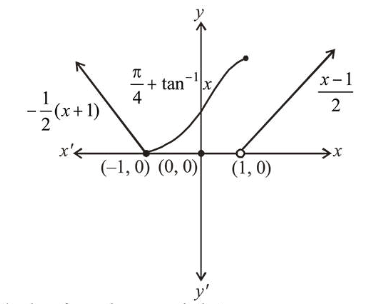# The functionQuestion:

The function $f(x)=\left\{\begin{array}{l}\frac{\pi}{4}+\tan ^{-1} x,|x| \leq 1 \\ \frac{1}{2}(|x|-1),|x|>1\end{array}\right.$ is :

1. (1) continuous on $\mathbf{R}-\{1\}$ and differentiable on $\mathbf{R}-\{-1,1\} .$

2. (2) both continuous and differentiable on $\mathbf{R}-\{1\}$.

3. (3) continuous on $\mathbf{R}-\{-1\}$ and differentiable on $\mathbf{R}-\{-1,1\} .$

4. (4) both continuous and differentiable on $\mathbf{R}-\{-1\}$.

Correct Option: 1

Solution:

$f(x)= \begin{cases}\frac{-x-1}{2}, & x<-1 \\ \frac{\pi}{4}+\tan ^{-1} x, & -1 \leq x \leq 1 \\ \frac{1}{2}(x-1), & x>1\end{cases}$It is clear from above graph that, $f(x)$ is discontinuous at $x=1$.

i.e. continuous on $R-\{1\}$

$f(x)$ is non-differentiable at $x=-1,1$

i.e. differentiable on $R-\{-1,1\}$.